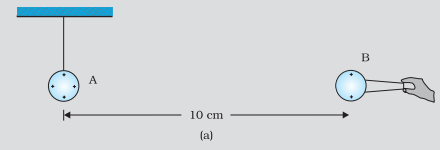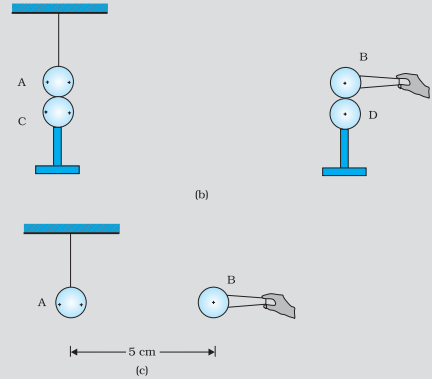Home/Class 12/Physics/

A charged metallic sphere $$A$$ is suspended by a nylon thread. Another charged metallic sphere $$B$$ held by an insulating handle is brought close to $$A$$ such that the distance between their centres is $$10\text{ cm }$$ , as shown in figure. The resulting repulsion of $$A$$ is noted (for example, by shining a beam of light and measuring the deflection of its shadow on a screen). Spheres $$A$$ and $$B$$ are touched by uncharged spheres $$C$$ and $$D$$ respectively, as shown in figure.$$C$$ and $$D$$ are then removed and $$B$$ is brought closer to $$A$$ to a distance of $$5.0\text{ cm }$$ between their centres, as shown in figure. What is the expected repulsion of $$A$$ on the basis of Coulomb’s law? Spheres $$A$$ and $$C$$ and spheres $$B$$ and $$D$$ have identical sizes. Ignore the sizes of $$A$$ and $$B$$ in comparison to the separation between their centres.Speed
00:00
06:19## QuestionPhysicsClass 12

A charged metallic sphere $$A$$ is suspended by a nylon thread. Another charged metallic sphere $$B$$ held by an insulating handle is brought close to $$A$$ such that the distance between their centres is $$10\text{ cm }$$ , as shown in figure. The resulting repulsion of $$A$$ is noted (for example, by shining a beam of light and measuring the deflection of its shadow on a screen). Spheres $$A$$ and $$B$$ are touched by uncharged spheres $$C$$ and $$D$$ respectively, as shown in figure.$$C$$ and $$D$$ are then removed and $$B$$ is brought closer to $$A$$ to a distance of $$5.0\text{ cm }$$ between their centres, as shown in figure. What is the expected repulsion of $$A$$ on the basis of Coulomb’s law? Spheres $$A$$ and $$C$$ and spheres $$B$$ and $$D$$ have identical sizes. Ignore the sizes of $$A$$ and $$B$$ in comparison to the separation between their centres.Let us consider the actual charge on sphere $$A$$ is $$q$$ and that on $$B$$ is $$q^\prime$$
The distance between the centres of $$A$$ and $$B$$ is $$r$$
Then the magnitude of the electrostatic force $$F$$ on each sphere $$A$$ and $$B$$ is given by
$$F=\frac 1{4\pi \varepsilon _0}\frac{\mathit{qq}^\prime }{r^2}$$
where as the sizes of the two spheres $$A$$ and $$B$$ are negligible here when compared to the distance $$r$$ .
As shown in figure, an identical but uncharged sphere $$C$$ touches $$A$$ and then the charges redistribute on $$A$$ and $$C$$ and, by symmetry, the actual charge $$q$$ on $$A$$ is redistributed between $$A$$ and $$C$$ . Now each sphere carries a charge of $$\dfrac{q}{2}.$$
Same way, after $$D$$ touches $$B$$ , the redistributed charge on each sphere becomes $$\dfrac{q^\prime}{2}.$$
As per the given data, after the spheres $$C$$ and $$D$$ are removed, the distance between the spheres $$A$$ and $$B$$ becomes halved.
Then the magnitude of the electrostatic force $$F^\prime$$ on each sphere is,
$$F^\prime =\frac 1{4\pi \varepsilon _0}\frac{\left(\frac q 2\right)\left(\frac{q^\prime } 2\right)}{(\frac{r}{2})^2}=\frac 1{4\pi \varepsilon _0}\frac{\mathit{qq}^\prime }{r^2}=F$$
This reveals that the electrostatic force on $$A$$ due to $$B$$ remains unaltered as $$F=F^\prime .$$